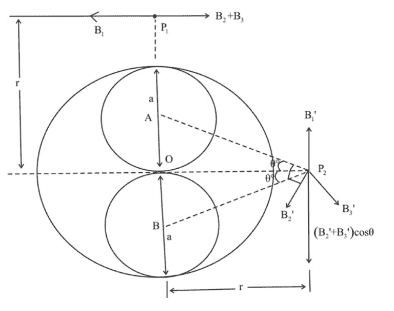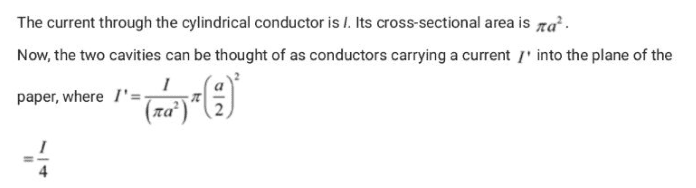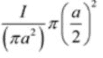# Cylindrical Conductors Carrying a Current I -- Formula (?)

requied
Homework Statement:
The current through the cylindrical conductor s I. Its cross sectional area is pi.a^2. Now, the two cavities on the figure above can be thought of as conductors carrying a current I' into the plane of the paper, where;
Relevant Equations:
I'= [I/(pi.a^2)].pi.[a/2]^2How can I' be the formula above? Is there any formula to get this sameHomework Helper
The suggested path is to treat the situation as if you had a full current in the entire big conductor without the cavities, and then add in the effect of having an additional current going the other way where the cavities should be.

Did you try that?

IRL the current would be on the outer surface of the conductor ... it looks like you are supposed to model the current as uniform through the conductor (check).

Note: you can use TeX markup for equations ...

$$\frac{1}{\pi a^2}\pi \left(\frac{a}{2}\right)^2 = \frac{1}{4}$$

The code fopr that was
Code:
$$\frac{1}{\pi a^2}\pi \left(\frac{a}{2}\right)^2 = \frac{1}{4}$$